ISEE Middle Level Math : How to add fractions

Example Questions

Example Question #37 : How To Add Fractions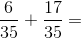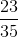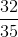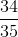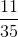Explanation:

Add the numerators and keep the denominator: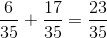Answer:Example Question #38 : How To Add Fractions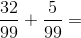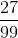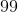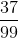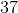Explanation:

Add the numerators and keep the denominator the same: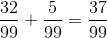Answer:Example Question #39 : How To Add Fractions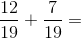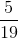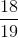Explanation:

Add the numerators and keep the denominator the same. Then, simplify: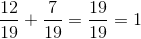Answer:Example Question #40 : How To Add Fractions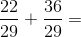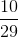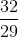Explanation:

Add the numerators and keep the denominator the same. Then, reduce: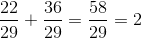Answer:Example Question #121 : Fractions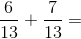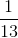Explanation:

Add the numerators and keep the denominator; then, reduce: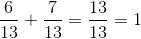Answer:Example Question #41 : How To Add Fractions

Solve: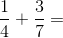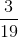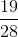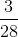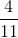Explanation:

The least common denominator ofandis.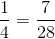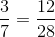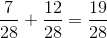Example Question #42 : How To Add Fractions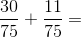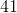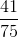Explanation:

Add the numerators and keep the denominator the same: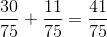Answer:Example Question #43 : How To Add Fractions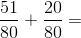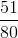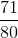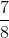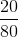Explanation:

Add the numerators and keep the denominator the same: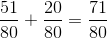Answer:Example Question #44 : How To Add Fractions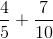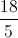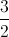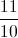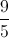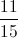Explanation:

First, we need to find a common denominator. In this case, we can convert both denominators to.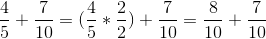Now we can add the numerators.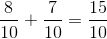Both the numerator and denominator are divisible by, so we can simplify.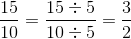Example Question #45 : How To Add Fractions

Add: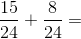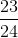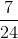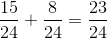Answer: